## Wednesday, April 29, 2015

### New version of phytools (phytools 0.4-56) now on CRAN

A new phytools version (phytools 0.4-56) is now on CRAN. As of writing, Mac & Windows binaries had not yet been built. Hopefully those will be available, and will begin to percolate through the mirror repositories, within a few days.

Among the updates in this version are the following:

(1) A bug fix in `plotTree.singletons` to permit multifurcations.

(2) Multiple fixes to the function `reroot` for trees with node labels (1, 2, 3).

(3) A new interactive tree plotter (`collapseTree`) to collapse & expand a fan-style phylogeny (1, 2, 3).

(4) A new version of the `biplot` method for objects of class `"phyl.pca"` to permit choice in the PC axes to be plotted (here).

(5) A kindly user-supplied bug fix for `fastAnc`.

(6) A fix in how the environmental variable `"last_plot.phylo"` is created by `plotSimmap` (here).

(7) Updates to a wide range of phytools plotting functions to permit smoother plotting and animation.

Finally, (8) major update to `plotTree.wBars` to permit tip labels to be plotted (here).

In addition to this non-comprehensive list of phytools updates, I also made many changes and additions to the phytools manual pages for the present CRAN release.

There were a few different functions described on the phytools blog that have not been added to this package update but may feature in a future version of phytools.

For example:

(1) A function to identify the youngest node on the tree with N or more descendant tips (here).

(2) A function to simulate random branch lengths under a pure-birth (Yule process) conditioned on a user-supplied tree topology (1, also see 2).

(3) A function to convert a phylogeny with node labels to a taxonomy (here).

Finally, (4) a function to split a plotted tree across multiple plotting devices or pages (1, 2).

Obviously, the latest version of phytools can be obtained from phytools.org as well as from the phytools CRAN page.

Here is a previously posted video showing use of the phytools interactive tree pruner, `collapseTree`:

## Tuesday, April 28, 2015

### plotTree.wBars with tip labels

I have just submitted a new version of phytools (0.4-56) to CRAN. Fingers crossed that it survives scrutiny & is accepted; however in the meantime it can be downloaded from the phytools page.

In a different post I will detail the updates (which are many) from both the previous CRAN version and from other recent non-CRAN phytools releases; however one feature that has been added is a modification to the phytools function `plotTree.wBars` to permit tip labels to be plotted. This function adds bars for a continuous trait to the tips of the tree. Here is a quick demo with the default conditions:

``````library(phytools)
packageVersion("phytools")
``````
``````##  '0.4.56'
``````
``````## make realistic looking tip labels
tip.label<-replicate(60,paste(sample(LETTERS,1),"._",
paste(sample(letters,round(runif(n=1,min=4,max=8))),
collapse=""),sep=""))
## simulate tree
tree<-pbtree(n=60,tip.label=tip.label,scale=1)
## simulate positive trait data - negative values are
## permitted, and I will show that below
x<-abs(fastBM(tree))
## finally, plot without tree labels
plotTree.wBars(tree,x,scale=0.2)
``````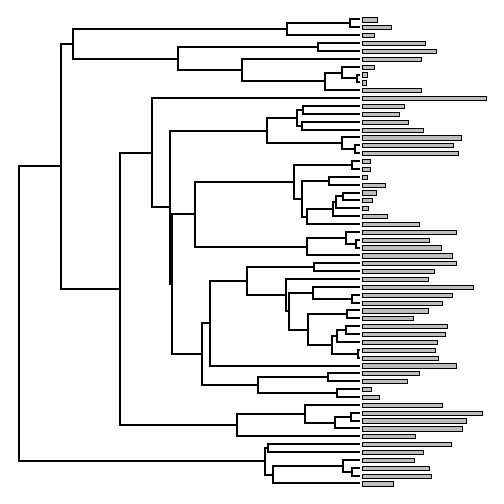Although I received lots of positive feedback about this function, users wanted to be able to include tip labels (very reasonably, I must admit). The only added complication with this is that we now have to compute not only how much space we need for the phylogeny & the trait data to be plotted, but also how much space is required to plot the tip labels. This is more complicated that it sounds, even, because the amount of space required for the tip labels cannot be found without first creating the plotting device.

The code for the updated version of `plotTree.wBars` is here.

Here is how it looks, for the same data & tree:

``````plotTree.wBars(tree,x,scale=0.2,tip.labels=TRUE,fsize=0.6)
``````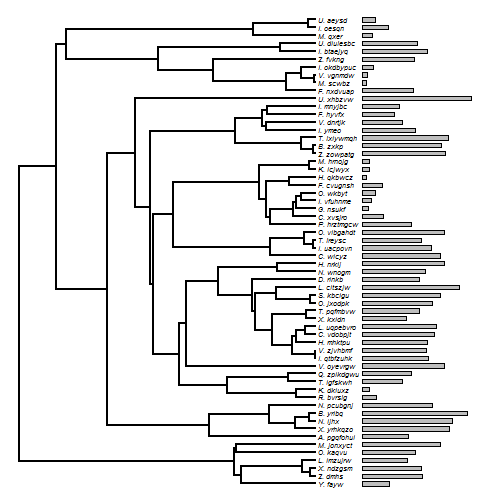For larger trees especially, we may want to plot our tree in a fan style. This also works with tip labels, for instance as follows:

``````tip.label<-replicate(200,paste(sample(LETTERS,1),"._",
paste(sample(letters,round(runif(n=1,min=4,max=8))),
collapse=""),sep=""))
tree<-pbtree(n=200,tip.label=tip.label,scale=1)
x<-abs(fastBM(tree))
plotTree.wBars(tree,x,fsize=0.4,scale=0.1,tip.labels=TRUE,type="fan",lwd=1)
``````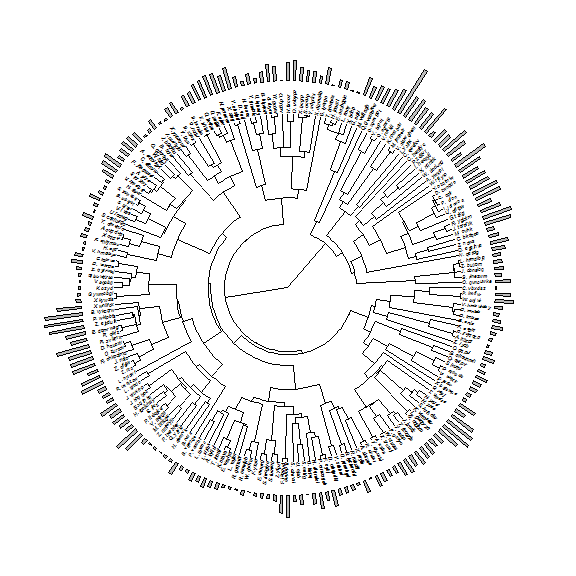I'm sure I've demonstrated this before, but it is fairly straightforward to combine `plotTree.wBars` with other phytools plotting methods, such as `contMap` - continuous character mapping onto the tree. So for example:

``````## create an object of class "contMap"
obj<-contMap(tree,x,plot=FALSE)
plotTree.wBars(obj\$tree,x,fsize=0.4,scale=0.1,tip.labels=TRUE,
type="fan",method="plotSimmap",colors=obj\$cols)
prompt=FALSE,x=0.9*par()\$usr,y=0.9*par()\$usr)
``````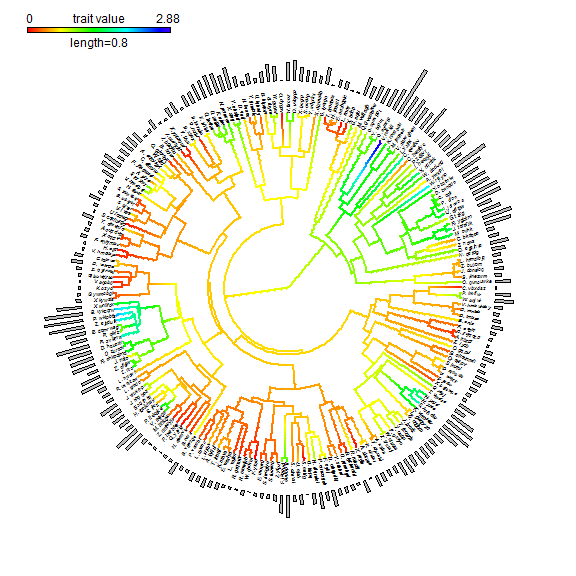You might notice lots of 'aliasing' in this plot. Obviously, we wouldn't want this for publication. We can easily avoid it by exporting to PDF or other lossless formats from within R. In this case, we could simply do:

``````pdf(file="28Apr15-post.pdf")
plotTree.wBars(obj\$tree,x,fsize=0.4,scale=0.1,tip.labels=TRUE,
type="fan",method="plotSimmap",colors=obj\$cols)
prompt=FALSE,x=0.9*par()\$usr,y=0.9*par()\$usr)
dev.off()
``````
``````## png
##   2
``````

You can see this product here: 28Apr15-post.pdf.

Finally, we can plot negative values. So, again with a smaller tree:

``````tip.label<-replicate(60,paste(sample(LETTERS,1),"._",
paste(sample(letters,round(runif(n=1,min=4,max=8))),
collapse=""),sep=""))
tree<-pbtree(n=60,tip.label=tip.label,scale=1)
x<-fastBM(tree)
plotTree.wBars(tree,x,scale=0.2,tip.labels=TRUE,fsize=0.6)
``````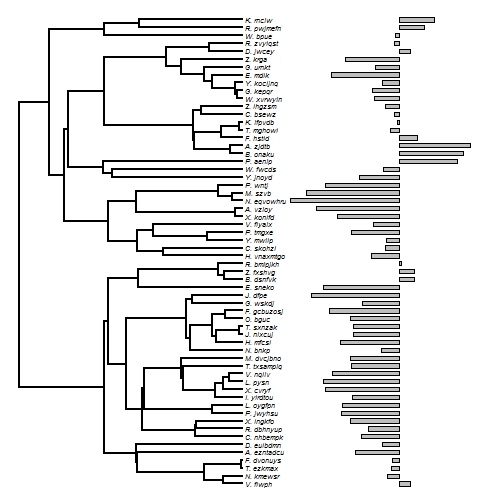``````## or
obj<-contMap(tree,x,plot=FALSE)
plotTree.wBars(obj\$tree,x,fsize=0.6,scale=0.2,tip.labels=TRUE,
method="plotSimmap",colors=obj\$cols)
``````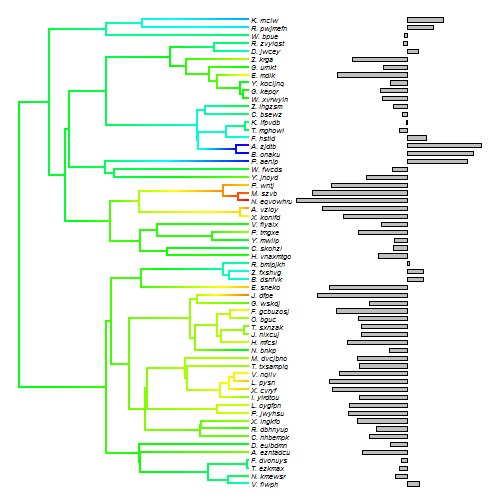OK, that's it.